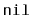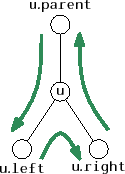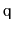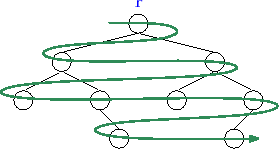Subsections

# 6.1 BinaryTree: A Basic Binary Tree

The simplest way to represent a node,, in a binary tree is to store the (at most three) neighbours ofexplicitly:

```    class BTNode<Node extends BTNode<Node>> {
Node left;
Node right;
Node parent;
}
```
When one of these three neighbours is not present, we set it to. In this way, external nodes in the tree as well as the parent of the root correspond to the value.

The binary tree itself can then be represented by a reference to its root node,:

```    Node r;
```

We can compute the depth of a node,, in a binary tree by counting the number of steps on the path fromto the root:

```    int depth(Node u) {
int d = 0;
while (u != r) {
u = u.parent;
d++;
}
return d;
}
```

## 6.1.1 Recursive Algorithms

It is very easy to compute facts about binary trees using recursive algorithms. For example, to compute the size of (number of nodes in) a binary tree rooted at node, we recursively compute the sizes of the two subtrees rooted at the children of, sum these sizes, and add one:

```    int size(Node u) {
if (u == nil) return 0;
return 1 + size(u.left) + size(u.right);
}
```

To compute the height of a nodewe can compute the height of's two subtrees, take the maximum, and add one:

```    int height(Node u) {
if (u == nil) return -1;
return 1 + Math.max(height(u.left), height(u.right));
}
```

## 6.1.2 Traversing Binary Trees

The two algorithms from the previous section use recursion to visit all the nodes in a binary tree. Each of them visits the nodes of the binary tree in the same order as the following code:

```    void traverse(Node u) {
if (u == nil) return;
traverse(u.left);
traverse(u.right);
}
```

Using recursion this way produces very short, simple code, but can be problematic. The maximum depth of the recursion is given by the maximum depth of a node in the binary tree, i.e., the tree's height. If the height of the tree is very large, then this could very well use more stack space than is available, causing a crash.

Luckily, traversing a binary tree can be done without recursion. This is done using an algorithm that uses where it came from to decide where it will go next. See Figure 6.3. If we arrive at a nodefrom, then the next thing to do is to visit. If we arrive atfrom, then the next thing to do is to visit. If we arrive atfrom, then we are done visiting's subtree, so we return to. The following code implements this idea, with code included for handling the cases where any of,, oris:

```    void traverse2() {
Node u = r, prev = nil, next;
while (u != nil) {
if (prev == u.parent) {
if (u.left != nil) next = u.left;
else if (u.right != nil) next = u.right;
else next = u.parent;
} else if (prev == u.left) {
if (u.right != nil) next = u.right;
else next = u.parent;
} else {
next = u.parent;
}
prev = u;
u = next;
}
}
```

Figure 6.3: The three cases that occur at nodewhen traversing a binary tree non-recursively, and the resulting traversal of the tree.The same things that can be computed with recursive algorithms can also be done this way. For example, to compute the size of the tree we keep a counter,, and incrementwhenever visiting a node for the first time:

```    int size2() {
Node u = r, prev = nil, next;
int n = 0;
while (u != nil) {
if (prev == u.parent) {
n++;
if (u.left != nil) next = u.left;
else if (u.right != nil) next = u.right;
else next = u.parent;
} else if (prev == u.left) {
if (u.right != nil) next = u.right;
else next = u.parent;
} else {
next = u.parent;
}
prev = u;
u = next;
}
return n;
}
```

In some implementations of binary trees, thefield is not used. When this is the case, a non-recursive implementation is still possible, but the implementation has to use a List (or Stack) to keep track of the path from the current node to the root.

A special kind of traversal that does not fit the pattern of the above functions is the breadth-first traversal. In a breadth-first traversal, the nodes are visited level-by-level starting at the root and working our way down, visiting the nodes at each level from left to right. This is similar to the way we would read a page of English text. (See Figure 6.4.) This is implemented using a queue,, that initially contains only the root,. At each step, we extract the next node,, from, processand addand(if they are non-) to:

```    void bfTraverse() {